# Android撸一个转盘抽奖

## 前言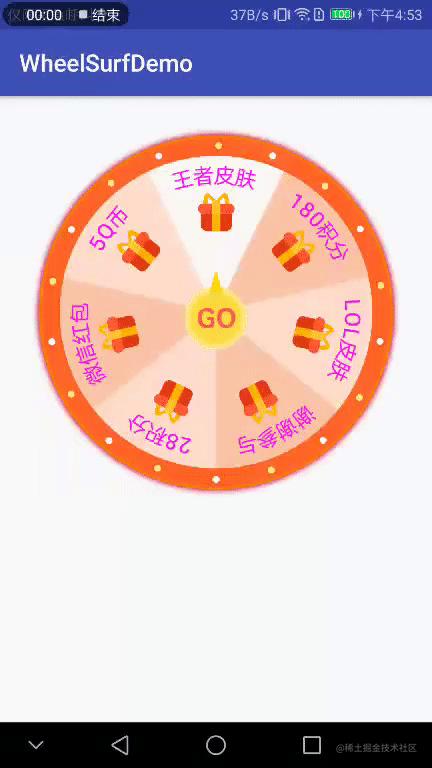DEMO效果

## 具体实现思路

#### 第一种傻瓜式思路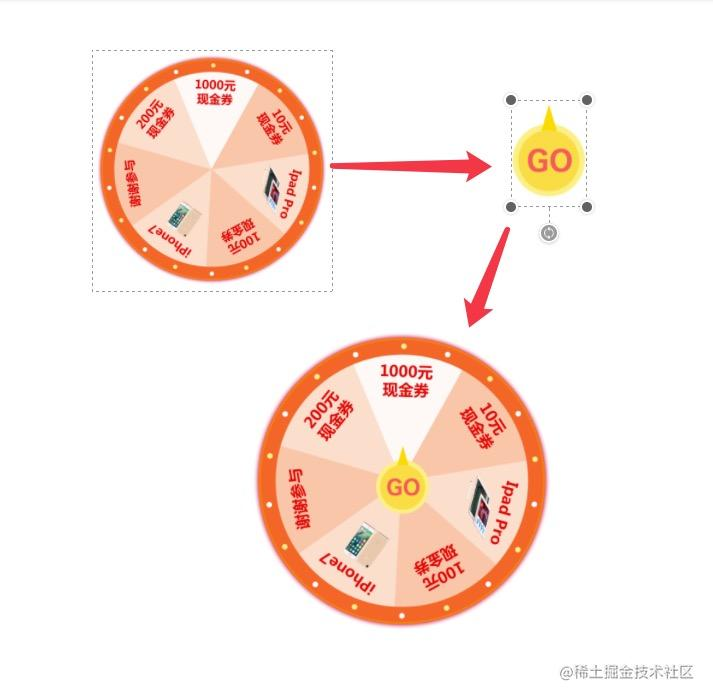傻瓜式操作

#### WheelSurfPanView的实现

• 分区和绘制背景
这里的分区指的是将圆盘等额分成多少个扇形
• 绘制文字
扇形分好之后，我们需要在每个扇形上面绘制文字描述
• 绘制图片
扇形分好之后，我们还需要在每个扇形上面绘制图片说明
• 盖上最外层的圈圈
扇形部分处理好了之后，我们需要在最外层盖上一个圆环，这样才叫转盘
• 旋转自己
绘制完一切之后，它还需要等待指令旋转自己

#### 重点，敲黑板

``````float startAngle = - mAngle / 2 - 90;

``````    // 计算初始角度
// 从最上面开始绘制扇形会好看一点 mAngle指的是每一个扇形的角度
float startAngle = - mAngle / 2 - 90;
for ( int i = 0; i < mTypeNum; i++ ) {
//设置绘制时画笔的颜色
mPaint.setColor(mColors[i]);
//画一个扇形 指定范围 范围就是整个圆盘的大小
//第一个参数 oval 参数的作用是：定义的圆弧的形状和大小的范围
//第二个参数：float startAngle 这个参数的作用是设置圆弧是从哪个角度来顺时针绘画的
//第三个参数：float sweepAngle 这个参数的作用是设置圆弧扫过的角度
//第四个参数：boolean useCenter 这个参数的作用是设置我们的圆弧在绘画的时候，是否经过圆形值得注意的是，这个参数在我们的 mPaint.setStyle(Paint.Style.STROKE); 设置为描边属性的时候，是看不出效果的。
//第五个参数：Paint paint 画笔
canvas.drawArc(rect, startAngle, mAngle, true, mPaint);
//重置开始角度
startAngle = startAngle + mAngle;
}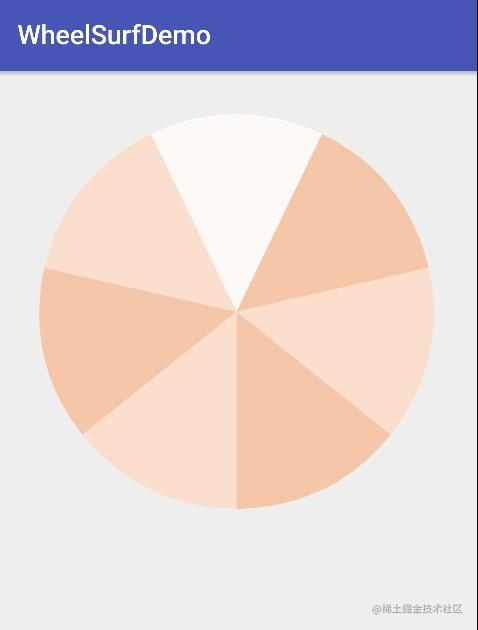分区效果

``````    float startAngle = -mAngle / 2 - 90;

for ( int i = 0; i < mTypeNum; i++ ) {

//重置开始角度
startAngle = startAngle + mAngle;
}

//绘制文字
private void drawText(float startAngle, String string, int radius, Paint textPaint, Canvas canvas) {
//创建绘制路径
Path circlePath = new Path();
//范围也是整个圆盘
//给定扇形的范围

//圆弧的水平偏移  与路径起始点的水平偏移距离
float textWidth = textPaint.measureText(string);
//圆弧的垂直偏移  与路径中心的垂直偏移量
float hOffset = ( float ) (Math.sin(mAngle / 2 / 180 * Math.PI) * radius) - textWidth / 2;

//绘制文字
canvas.drawTextOnPath(string, circlePath, hOffset, radius / 4, textPaint);
}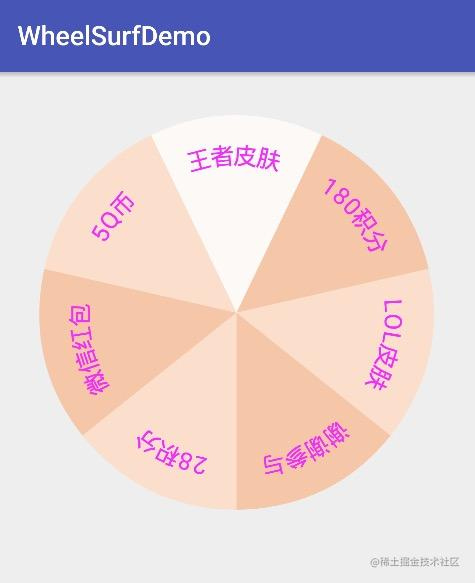绘制文字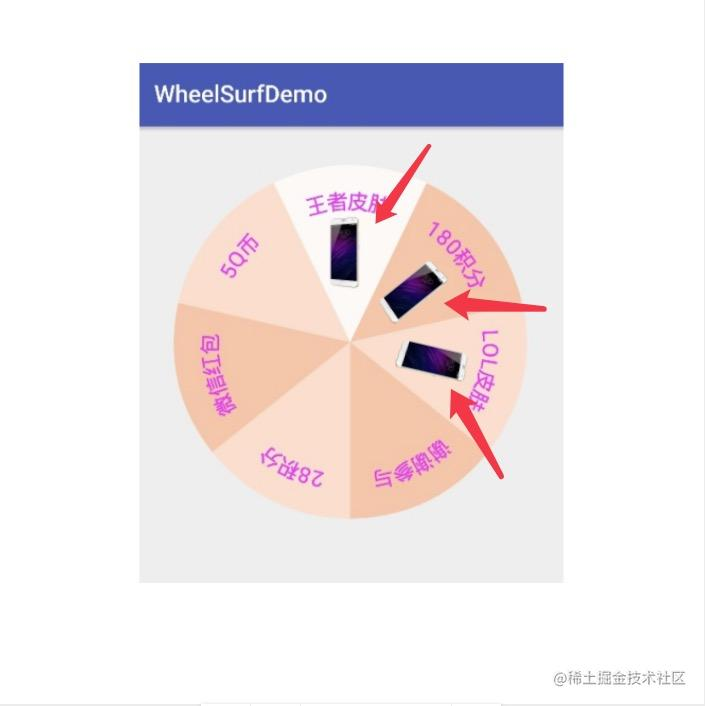图片旋转后的效果

``````//加载分类图片 存放图片的集合
mListBitmap = new ArrayList<>();
for ( int i = 0; i < mTypeNum; i++ ) {
Bitmap bitmap = BitmapFactory.decodeResource(context.getResources(), mIcons[i]);
int ww = bitmap.getWidth();
int hh = bitmap.getHeight();
// 定义矩阵对象
Matrix matrix = new Matrix();
// 缩放原图
matrix.postScale(1f, 1f);
// 向左旋转mAngle度，参数为正则向右旋转
matrix.postRotate(mAngle * i);
//bmp.getWidth(), 500分别表示重绘后的位图宽高
Bitmap dstbmp = Bitmap.createBitmap(bitmap, 0, 0, ww, hh,matrix, true);
}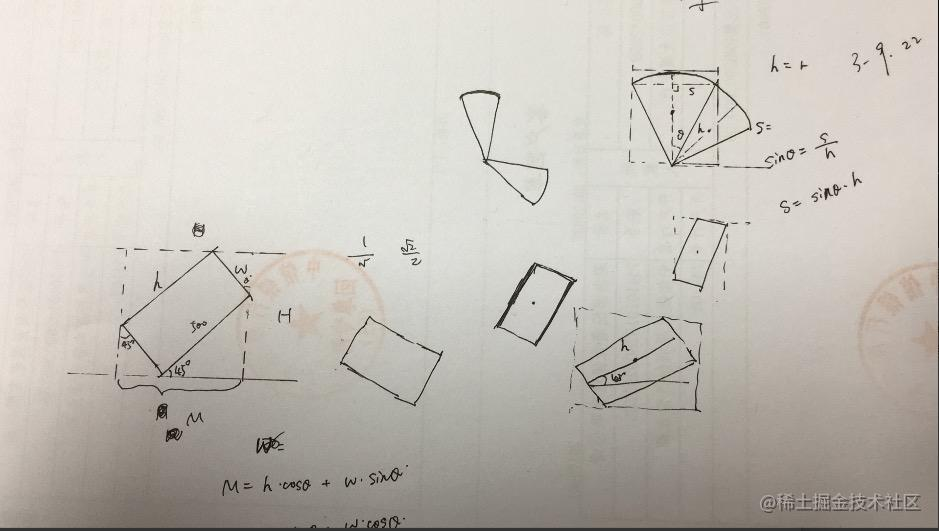草稿

``````   float startAngle = -mAngle / 2 - 90;
//这些值是为了精确图片的位置

for ( int i = 0; i < mTypeNum; i++ ) {
int imgWidth = mRadius / 4;
float angle = ( float ) Math.toRadians(startAngle + mAngle / 2);
//确定图片在圆弧中 中心点的位置
float x = ( float ) (width / 2 + (mRadius / 2 + mRadius / 12) * Math.cos(angle));
float y = ( float ) (height / 2 + (mRadius / 2 + mRadius / 12) * Math.sin(angle));
// 确定绘制图片的位置
RectF rect1 = new RectF(x - imgWidth / 2, y - imgWidth / 2, x + imgWidth / 2, y + imgWidth / 2);
canvas.drawBitmap(mListBitmap.get(i), null, rect1, null);
//重置开始角度
startAngle = startAngle + mAngle;
}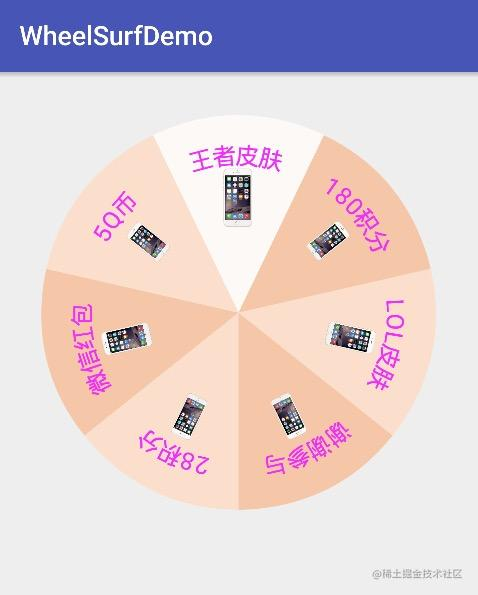绘制图片后

`````` mTextPaint.setColor(Color.WHITE);
canvas.drawRect(rect1, mTextPaint);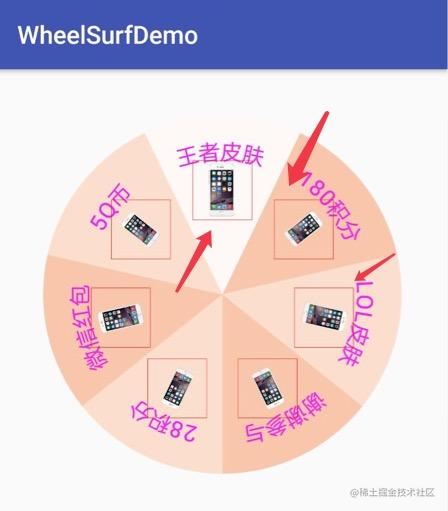带边框的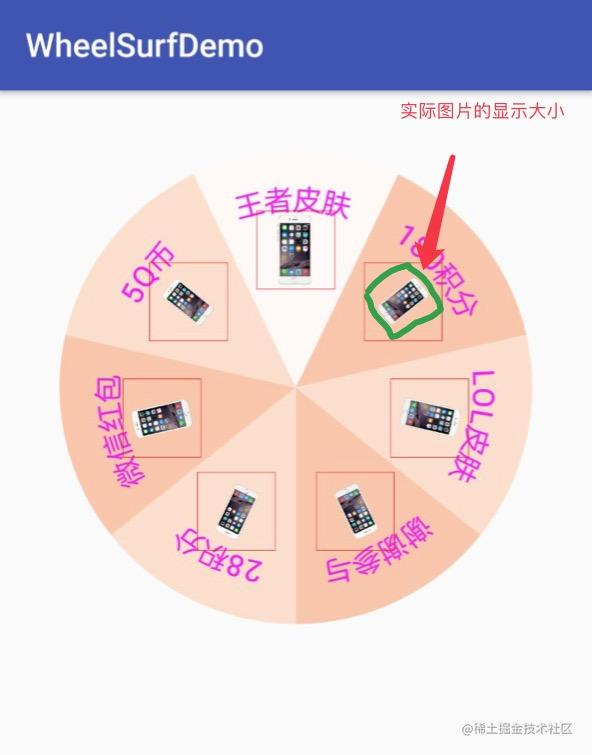图片实际大小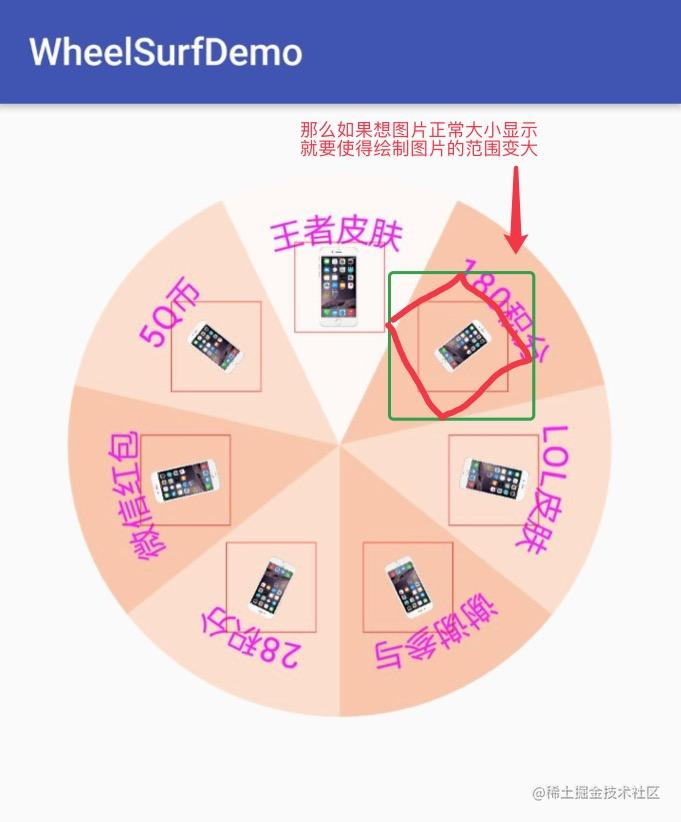实际需要的大小

``````  // 从最上面开始绘制扇形会好看一点
float startAngle = -mAngle / 2 - 90;
//这些值是为了精确图片的位置
for ( int i = 0; i < mTypeNum; i++ ) {
//设置绘制时画笔的颜色
mPaint.setColor(mColors[i]);
//画一个扇形
canvas.drawArc(rect, startAngle, mAngle, true, mPaint);
mTextPaint.setColor(mTextColor);

int imgWidth = mRadius / 3;
//计算实际宽高
int w = ( int ) (Math.abs(Math.cos(Math.toRadians(Math.abs(180 - mAngle * i)))) *
imgWidth + imgWidth * Math.abs(Math.sin(Math.toRadians(Math.abs(180 - mAngle * i)))));
int h = ( int ) (Math.abs(Math.sin(Math.toRadians(Math.abs(180 - mAngle * i)))) *
imgWidth + imgWidth * Math.abs(Math.cos(Math.toRadians(Math.abs(180 - mAngle * i)))));

float angle = ( float ) Math.toRadians(startAngle + mAngle / 2);

//确定图片在圆弧中 中心点的位置
float x = ( float ) (width / 2 + (mRadius / 2 + mRadius / 12) * Math.cos(angle));
float y = ( float ) (height / 2 + (mRadius / 2 + mRadius / 12) * Math.sin(angle));
// 确定绘制图片的位置
RectF rect1 = new RectF(x - w / 2, y - h / 2, x + w / 2, y + h / 2);
canvas.drawBitmap(mListBitmap.get(i), null, rect1, null);

//重置开始角度
startAngle = startAngle + mAngle;
}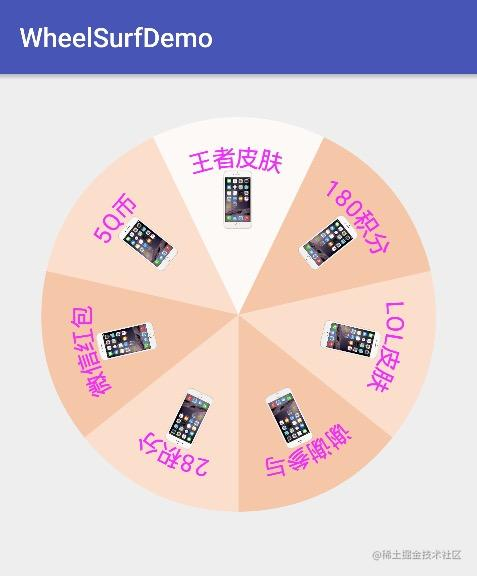jjjjjjjjjjjj.png

``````   //最后绘制圆环
Rect mDestRect = new Rect(0, 0, mWidth, mWidth);
canvas.drawBitmap(mYuanHuan, null, mDestRect, mPaint);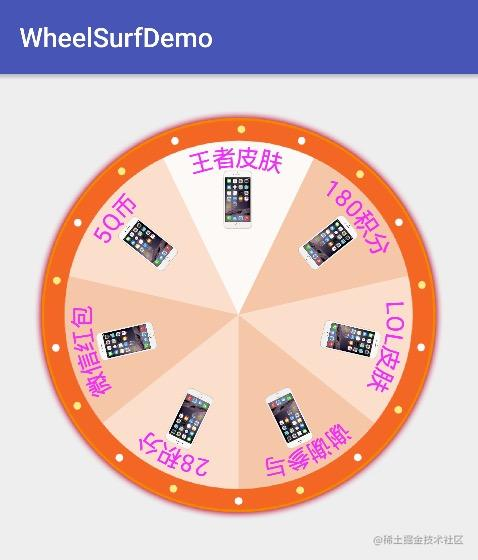加上圆圈

``````/**
* 开始转动
* pos 位置 1 开始 这里的位置上是按照逆时针递增的 比如当前指的那个选项是第一个  那么他左边的那个是第二个 以此类推
*/
public void startRotate(final int pos) {
//最低圈数是mMinTimes圈
int newAngle = ( int ) (360 * mMinTimes + (pos - 1) * mAngle + currAngle - (lastPosition == 0 ? 0 : ((lastPosition - 1) * mAngle)));
//计算目前的角度划过的扇形份数
int num = ( int ) ((newAngle - currAngle) / mAngle);
ObjectAnimator anim = ObjectAnimator.ofFloat(WheelSurfPanView.this, "rotation", currAngle, newAngle);
currAngle = newAngle;
lastPosition = pos;
// 动画的持续时间，执行多久？
anim.setDuration(num * mVarTime);
@Override
public void onAnimationUpdate(ValueAnimator animation) {
//将动画的过程态回调给调用者
if ( rotateListener != null )
rotateListener.rotating(animation);
}
});
@Override
public void onAnimationEnd(Animator animation) {
super.onAnimationEnd(animation);
//当旋转结束的时候回调给调用者当前所选择的内容
if ( rotateListener != null ) {
if ( mType == 1 ) {
//去空格和前后空格后输出
String des = mDeses[(mTypeNum - pos + 1) %
mTypeNum].trim().replaceAll(" ", "");
rotateListener.rotateEnd(pos, des);
} else {
rotateListener.rotateEnd(pos, "");
}
}
}
});
// 正式开始启动执行动画
anim.start();
}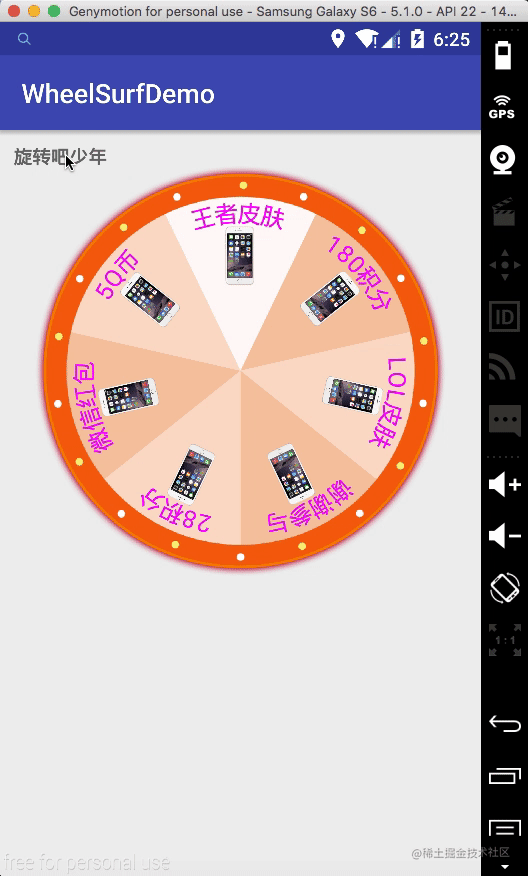旋转自己

#### WheelSurfView的实现

``````package com.cretin.www.wheelsurfdemo.view;

import android.annotation.TargetApi;
import android.content.Context;
import android.content.res.TypedArray;
import android.os.Build;
import android.util.AttributeSet;
import android.view.View;
import android.view.ViewGroup;
import android.view.ViewTreeObserver;
import android.widget.ImageView;
import android.widget.RelativeLayout;

import com.cretin.www.wheelsurfdemo.R;
import com.cretin.www.wheelsurfdemo.RotateListener;

/**
* Created by cretin on 2017/12/26.
*/

public class WheelSurfView extends RelativeLayout {
//当前的圆盘VIew
private WheelSurfPanView mWheelSurfPanView;
//Context
private Context mContext;
//开始按钮
private ImageView mStart;
//动画回调监听
private RotateListener rotateListener;

public void setRotateListener(RotateListener rotateListener) {
mWheelSurfPanView.setRotateListener(rotateListener);
this.rotateListener = rotateListener;
}

public WheelSurfView(Context context) {
super(context);
init(context, null);
}

public WheelSurfView(Context context, AttributeSet attrs) {
super(context, attrs);
init(context, attrs);
}

public WheelSurfView(Context context, AttributeSet attrs, int defStyleAttr) {
super(context, attrs, defStyleAttr);
init(context, attrs);
}

//开始抽奖的图标
private Integer mGoImgRes;

private void init(Context context, AttributeSet attrs) {
mContext = context;
if ( attrs != null ) {
//获得这个控件对应的属性。
TypedArray typedArray = getContext().obtainStyledAttributes(attrs, R.styleable.wheelSurfView);
try {
mGoImgRes = typedArray.getResourceId(R.styleable.wheelSurfView_goImg, 0);
} finally { //回收这个对象
typedArray.recycle();
}
}

//添加圆盘视图
mWheelSurfPanView = new WheelSurfPanView(mContext, attrs);
RelativeLayout.LayoutParams layoutParams =
new RelativeLayout.LayoutParams(ViewGroup.LayoutParams.MATCH_PARENT, ViewGroup.LayoutParams.MATCH_PARENT);
mWheelSurfPanView.setLayoutParams(layoutParams);

//添加开始按钮
mStart = new ImageView(mContext);
//如果用户没有设置自定义的图标就使用默认的
if ( mGoImgRes == 0 ) {
mStart.setImageResource(R.mipmap.node);
} else {
mStart.setImageResource(mGoImgRes);
}
//给图片设置LayoutParams
RelativeLayout.LayoutParams llStart =
new RelativeLayout.LayoutParams(ViewGroup.LayoutParams.WRAP_CONTENT, ViewGroup.LayoutParams.WRAP_CONTENT);
mStart.setLayoutParams(llStart);

mStart.setOnClickListener(new OnClickListener() {
@Override
public void onClick(View v) {
//调用此方法是将主动权交个调用者 由调用者调用开始旋转的方法
rotateListener.rotateBefore(( ImageView ) v);
}
});
}

/**
* 开始旋转
*
* @param pisition 旋转最终的位置 注意 从1 开始 而且是逆时针递增
*/
public void startRotate(int pisition) {
if ( mWheelSurfPanView != null ) {
mWheelSurfPanView.startRotate(pisition);
}
}

@Override
protected void onMeasure(int widthMeasureSpec, int heightMeasureSpec) {
//视图是个正方形的 所以有宽就足够了 默认值是500 也就是WRAP_CONTENT的时候
setMeasuredDimension(getDefaultSize(0, widthMeasureSpec), getDefaultSize(0, heightMeasureSpec));
// Children are just made to fill our space.
final int childWidthSize = getMeasuredWidth();
//高度和宽度一样
heightMeasureSpec = widthMeasureSpec = MeasureSpec.makeMeasureSpec(childWidthSize, MeasureSpec.EXACTLY);

//onMeasure调用获取到当前视图大小之后，
// 手动按照一定的比例计算出中间开始按钮的大小，
// 再设置给那个按钮，免得造成用户传的图片不合适之后显示贼难看
// 只设置一次
if ( isFirst ) {
isFirst = !isFirst;
//获取中间按钮的大小
ViewTreeObserver vto = mStart.getViewTreeObserver();
@TargetApi( Build.VERSION_CODES.KITKAT )
@Override
public void onGlobalLayout() {
mStart.getViewTreeObserver().removeGlobalOnLayoutListener(this);
float w = mStart.getMeasuredWidth();
float h = mStart.getMeasuredHeight();
//计算新的大小 默认为整个大小最大值的0.17 至于为什么是0.17  我只想说我乐意。。。。
int newW = ( int ) ((( float ) childWidthSize) * 0.17);
int newH = ( int ) ((( float ) childWidthSize) * 0.17 * h / w);
ViewGroup.LayoutParams layoutParams = mStart.getLayoutParams();
layoutParams.width = newW;
layoutParams.height = newH;
mStart.setLayoutParams(layoutParams);
}
});
}

super.onMeasure(widthMeasureSpec, heightMeasureSpec);
}

//记录当前是否是第一次回调onMeasure
private boolean isFirst = true;
}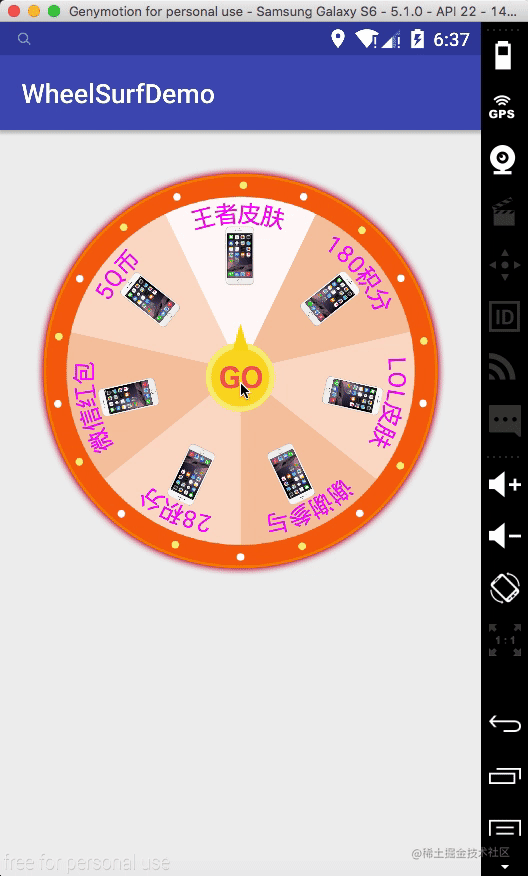最终效果

## 结语

• 直接给一张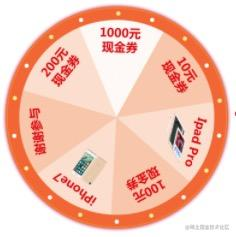直接给的圆底盘

• 使用第二种方式，给定分区数量，给定每个分区的文字描述和图标资源，给定每个分区的背景颜色，给定最外面大圈的素材，给定最中间的按钮素材，设置每个分区的旋转时间，设置最低的旋转圈数等等，最大化给调用者自定义的空间。

Android